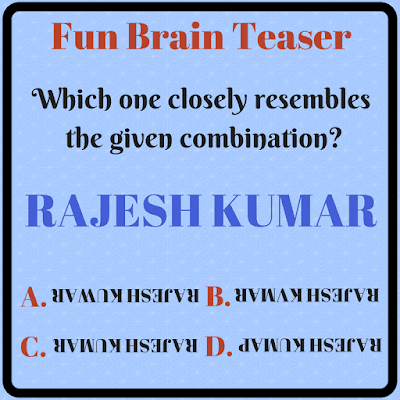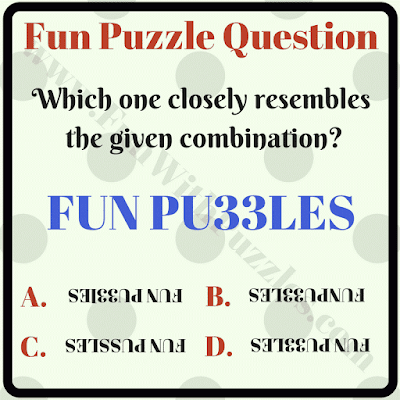Non-Verbal Reasoning is the ability to understand interpret and analyse visual information. There are many different types of puzzle questions to test non-verbal reasoning. In fact, there are many examinations in which non-verbal reasoning questions are being asked to test one's mental abilities.
Here are some non-verbal reasoning questions. In these puzzles, one-word containing alphabets and numbers are given. This word has been reversed in all puzzles. One has to choose the word which closely resembles the given word when reversed. There are four choices given in the answer and only one of the answer is correct. Let us see if you can solve these non-verbal reasoning questions quickly?1. Non-verbal reasoning easy question2. Non-verbal reasoning fun brain teaser3. Non verbal reasoning fun puzzle question4. Non-verbal reasoning hard question5. Non-verbal reasoning question to test your intelligence